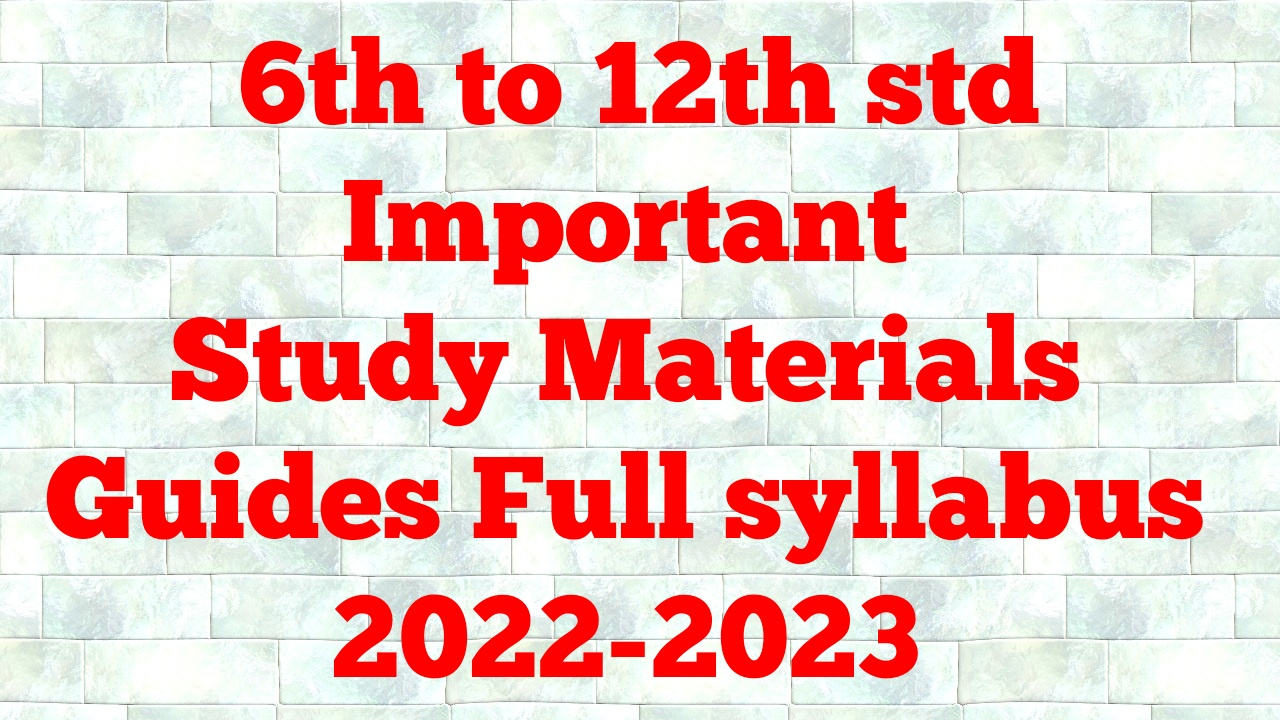# 12th Chemistry Refresher Course Unit 1 - Answer Key English Medium

### Basic concepts of Chemistry Answer key

Assessment:

1. How many water molecules are present in a drop of water weighing 0.018g?

1 mole of water=18g

0.018g------? moles

Number of moles=0.018/18=0.001 moles of water.

1 mole of water[ 18g ] contains 6.022 x 10²³ molecules .

0.001 moles contain ---------? molecules

=0..001x6.022 x 10²³

=6.022x10²⁰ molecules

∴The number of water molecules in a drop of water weighing 0.018 g is 6.022x10²⁰ molecules

2. Find the molar mass of Ethanol (C₂H₅OH) and Sucrose (C₁₂H₂₂O₁₁).

• Ethanol (C₂H₅OH)

Molar mass = (2 × 12) + (6 × 1) + (1 × 16)

= 24 + 6+16

= 46 g mol-1

• Sucrose (C₁₂H₂₂O₁₁)

Molar mass = (12 × 12) + (22 × 1) + (11 × 16)

= 144 + 22 + 176

= 342 g mol-1

3. Calculate the number of moles present in 646g of ammonia (NH3).

NH₃

molecular weight = 14+3×1=17

number of moles = gram weight÷molecular weight

= 646÷17

= 38 mol.

4. What is the mass of 1 gold atom (Au)?

The atomic mass of Gold = 197 g mol⁻¹.

197 g of gold contains 6.023 x 10²³ atoms of gold.

= 3.055 x 10²⁴ atoms of Gold.

5. If the equivalent mass of a trivalent metal element is 9g eq⁻¹, what will be the molar mass ofits anhydrous oxide?

Let the trivalent metal be M⁺³

Equivalent mass = mass of the metal / valance factor

9g eq⁻¹ = mass of the metal / 3 eq

Mass of the metal = 27 g

Oxide formed M₂O₃

Mass of the oxide = (2 × 27) + (3 × 16) = 102 g

1. வாசகர்களின் மேலான கருத்துக்களை அன்புடன் வரவேற்கின்றோம்.

 Send Your Materials This WhatsApp Number : 9095918266• 💯 10th Tamil Full Guides 2022-2023
• 💯 10th English Full Guide 2022-2023
• 💯 10th Maths Full Guide 2022-2023
• 💯 10th science Full Guide 2022-2023
• 💯 10th social full guide 2022-2023
• 💯 12th All subjects Full Guide study materials 2022-2023
• 💯 11th All subjects Full Guide study materials 2022-2023